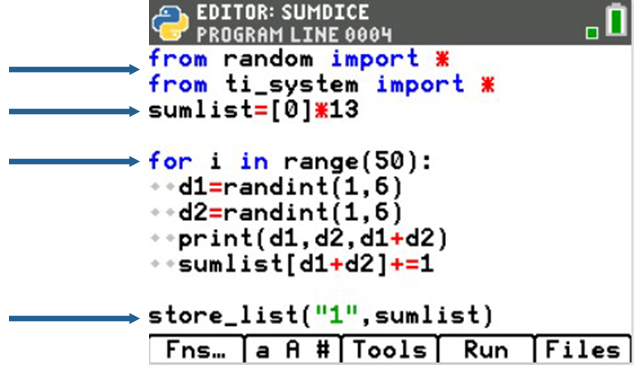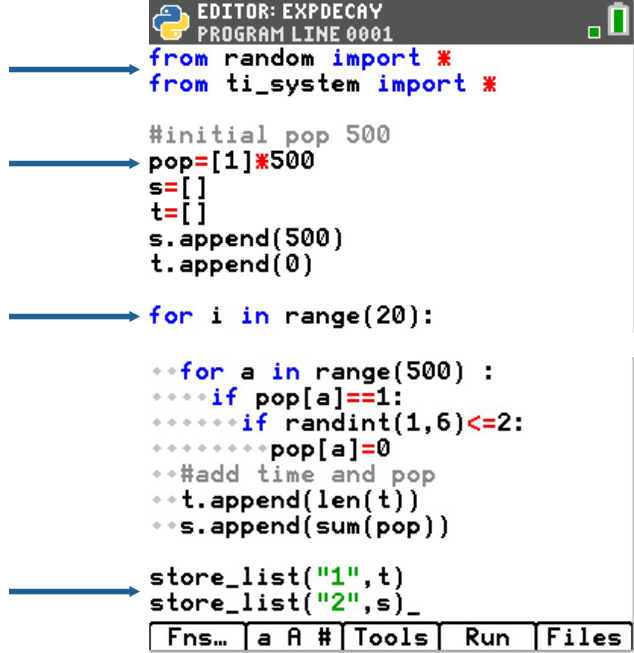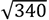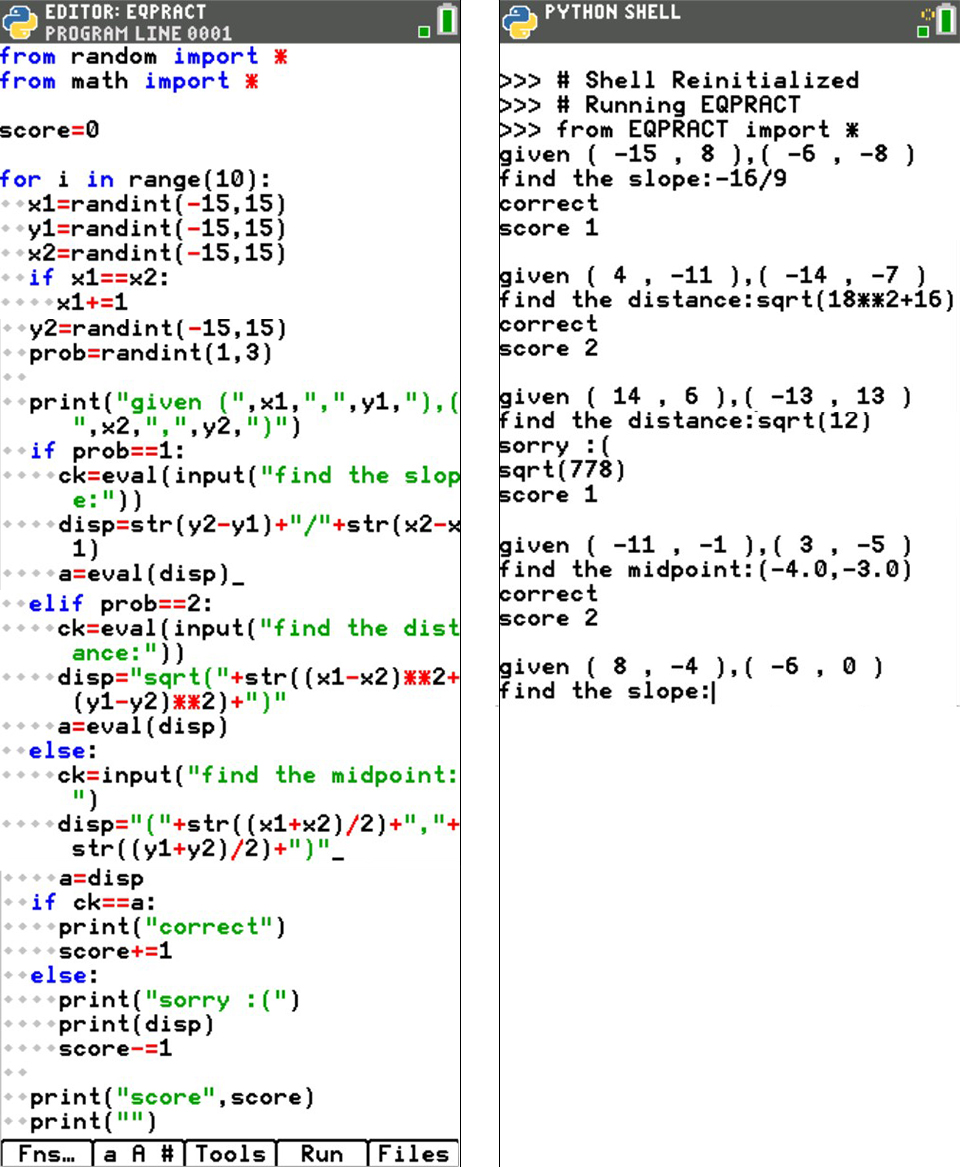## Using Python to Squeeze the Fun Back Into Math

Posted 07/16/2021 by Becky Byer

Have you heard the BIG news?
Python is now included in the new TI-84 Plus CE Python graphing calculator! What does this mean for math class? Python on the handheld gives teachers a tool to teach algorithmic thinking, provide authentic opportunities for problem solving, and enhance mathematical reasoning. Using coding to teach and explore mathematics uses all the math practice standards. Plus, when you teach students Python, you’ll be introducing them to one of the most widely used programming languages across a variety of industries.

You might be asking: Just how can coding be used to enhance math instruction?
Let’s look at one possible example: quadratics. We all teach it. What are some of the key ideas students should understand about quadratics?

• Quadratics can cross the x-axis twice, once or not at all.
• The quadratic formula is one way to calculate the x-intercepts.
• The discriminant can be used to determine if there are two distinct real x-intercepts, one double real x-intercept, or two imaginary x-intercepts.

By having your students code the Quadratic Project, they will use these three key math ideas as the foundation and will reinforce the concepts and ultimately provide the students with a tool to check their own work. Let’s take a look at the code, and see how it is used to sneak Python into exploring key math ideas:

• Quadratics can cross the x-axis twice, once or not at all. This leads to the three cases in the conditional statements below.

if

elif

else

• The discriminant defines the conditions.

disc = sqrt(b**2 – 4*a*c)

if disc > 0:

elif disc == 0:

else

• The quadratic formula is one way to calculate the x-intercepts. Use the quadratic formula and the discriminant in each of the three conditional statements to calculate the x-intercepts.

disc = b**2 – 4*a*c

if disc > 0:

r1 = (-b + sqrt(disc))/(2*a)

r2 = (-b – sqrt(disc))/(2*a)

elif disc == 0:

r1 = -b/(2*a)

r2 = r1

else

r1 = complex(-b,  sqrt(disc))/(2*a)

r2 = complex(-b,  -sqrt(disc))/(2*a)

See? That was easy! You just integrated coding into math.

Anyone thinking “wait, I can already code that using TI-Basic”? Don’t worry, TI-Basic is still on the TI-84 Plus CE Python graphing calculator, and you can still use it. But, you are going to want to give Python a shot. Trust me! Python now gives you even more powerful tools to enhance math instruction. Have no fear; even if you’ve never coded before, that is ok. Python is great for new coders, and we’ll talk resources in a bit.

So, what’s all the buzz about Python?

COLOR ME HAPPY! Python incorporates color in the editor. Keywords such as for, if and import are blue. Math operators such as =, +, * and are all red. Strings are encapsulated in “quotes” and appear in green. Comments following the # are a light gray. The color coding helps break up the code, find typos and draws attention to the different types of syntax. The tab indentions for ifs and loops stand out using the diamond symbol, ◊.

Python coding in the classroom is not an “add-in.” It is a tool that provides authentic opportunities to explore, look for patterns, use patterns and build learning. To reach all learners, we provide multimodal learning opportunities. Why would we not add coding to our multiple representation toolbox of physical, analytical, graphical, verbal and numerical representation?

Another excellent use of Python in the math classroom is simulations. In Python, lists are easily created, manipulated and imported or exported. The following two activities highlight examples of how Python could deepen math learning through simulations. Notice how the color makes the different components pop on the page.

Activity 1: Simulation Data Export and Probability

Python makes it easy to code simulation, then export the data to the TI-84 Plus CE Python system.

· In only nine short lines, students can roll a pair of dice 50 times and store the sum.

· The data is exported to L1 for use on the statplot and graph page.

 About the program Import the two libraries needed for the project. Create a list to store sums 0 through 12. (0 and 1 won’t be used.) Repeat the dice roll 50 times d1 equals a random number [1,6]. d1 equals a random number [1,6]. Print d1, d2, sum(useful for students to see the values). Add 1 to the count for the sum of that particular roll. Export the sumlist to L1.Project sample:In small groups, students could compare and contrast graphs. You could ask students:

· Are all sums equally likely?

· Which sums seem to have the highest probability?

· Using your data, what percent of dice rolls result in a sum of 9?

· Using your data, what percent of dice rolls result in a sum of 9 or more?

· Compare your answers above to those of your groups; do you all have similar results? Why or why not?

After a quick change in the loop range from 50 to 1000 roll, the group can check their hypothesis with the simulation.Now have students revisit the previous four questions.

· Did your answer stay the same? If not, which ones changed?

· What’s the probability for a sum of 2?

· What’s the probability for a sum of 8?

· Why do you think the probability for a sum of 2 is different than the sum of 8?

· If you changed the loop to 5000, do you think the probabilities would change or stay the same? Why?

With only a few modifications, students could explore different types and quantities of dice.

Activity 2: Data Export and Functions

When I taught algebra II, I used a hands-on probability activity to model exponential decay. At the beginning of class, I gave each student a six-sided dice and instructed them to stand. We’d play a game to see whose luck would hold the longest. Each round, students would roll the dice. If the dice showed a 1 or a 2, the student had to sit down. Each round, we recorded the number of students still standing. We played until everyone was seated. The students plotted the data on the calculator. We would then find a function to model the decay.

This class activity was a quick, high-energy simulation that explored exponential decay and modeling. While the exploration is interactive and uses classroom-collected data, it takes a lot of class time to generate the data. Python would be a great addition to extend learning. After collecting the data using real dice and students the first time, students could code the activity for further exploration individually or with small groups.

 About the program   The base code on the right models the class exploration with 500 students.   Import the two libraries needed for the project.   pop: list that has 500 people “standing” using 1s. s is the sum list that contains the count for each round. t keeps track of the round number. The first for loops create 20 rounds of the game. The second for loop looks at each “person” in the round. If the person is still standing, “1,” then roll the dice. If the dice is a 1 or 2, make the person sit, “0.” At the end of each round, add a round and the sum. Store the rounds list, t, in L1. Store the sum list, s, in L2.Students could then use the exported data to plot and model an exponential decay function.Now that students have the base code, each student/group could alter the code to match a new initial population and probability of sitting. Students could execute the code, reset the graph, then find an equation to model the situation.

With a few slight modifications, the same code could model exponential growth.

Another (and final) great use of Python: creating math review games
Over the years, I’ve found remedial through honors students have enjoyed making games to review math concepts. Python is perfect for this. Once coded, students can play their games as warmups, while waiting for others to complete a quiz, as part of class competitions, or even as a homework assignment. I’ve had students who won’t do an ounce of homework walk into class the next day, all of sudden excited and motivated to show me their high score from the night before on the game they coded themselves! If students have their own calculator, this empowers them to practice concepts as often as they choose.

The eval() function in Python lets students enter calculations as an input and store the result in a variable. In the example below, students do not need to knowto play the game. They can enter sqrt(18**2+16) as the input. The value is stored in a variable and used for comparison later.

Example review game

The game below lets students practice finding the distance, the slope and the midpoint given two points. Practiced individually in separate units, students do well with the different formulas. However, at the end of the semester, students can mix up formulas or misread the question. Creating a game such as this allows students to practice distinguishing between the three concepts.Reminder: If you are new to Python, no worries. Everything is menu driven which allows teachers and students to explore syntax and application. A great place to have students start to learn the basics of programming with Python is TI Codes: Python. The TI-84 Plus CE Python version is coming soon. Right now, you can check out the site for activities using Python on the TI-Nspire™ CX II graphing calculator.

Python in the math class doesn’t have to be “just one more thing” we teach

Python is a tool to teach the content we are already teaching in a manner that is meaningful and relevant to students. The examples above show how coding can reinforce content, allow students to look for and make sense out of patterns, reason abstractly, and construct learning. Let’s make this next school year full of enhanced learning using Python!

About the author: Becky Byer teaches math and computer science at Kelly Walsh High School in Casper, Wyoming. She is a Regional T³™ instructor, Nationally Board Certified and a reader for the AP® Computer Science Principles exam. She is passionate about integrating technology such as computer programming to build and enhance student understanding of mathematics.